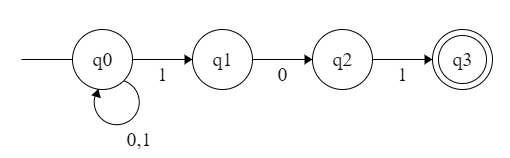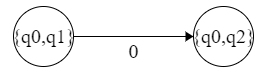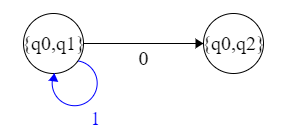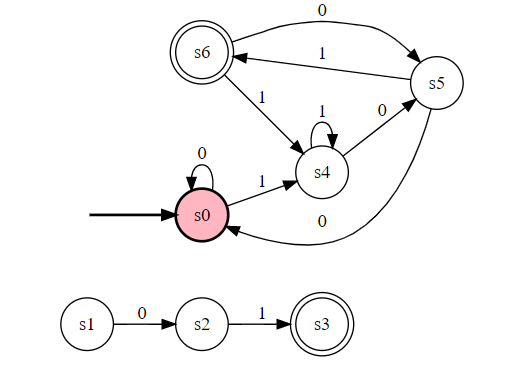# 自动机的幂集构造法详解

【例子】 这是识别 101 结尾的二进制串的 NFA。将其转换为 DFA。NFA 的状态转移表：

``````				0			1
->	{q0}		{q0}		{q0,q1}
{q1}		{q2}		/
{q2}		/			{q3}
*	{q3}		/			/
``````

• `{q0}` 跳至 `{q0}`

• `{q1}` 跳至 `{q2}```````				0			1
->	{q0}		{q0}		{q0,q1}
{q1}		{q2}		/
{q2}		/			{q3}
*	{q3}		/			/
{q0,q1}		{q0,q2}		{q0,q1}
``````

``````				0			1
->	{q0}		{q0}		{q0,q1}
{q1}		{q2}		/
{q2}		/			{q3}
*	{q3}		/			/
{q0,q1}		{q0,q2}		{q0,q1}
{q0,q2}		{q0}		{q0,q1,q3}
*	{q0,q1,q3}	{q0,q2}		{q0,q1}
``````

``````			0		1
->	s0		s0		s4
s1		s2		/
s2		/		s3
*	s3		/		/
s4		s5		s4
s5		s0		s6
*	s6		s5		s4
``````